• 在统计建模过程中往往会使用到采样技术，通过...简单随机抽样(SRS)是我们经常接触到的抽样方法，比如摸彩或抽奖，或办公室需要人出公差去送数据时用抽签决定人选。SRS的特色是母群体中的每一个体都相同的机会被选中
在统计建模过程中往往会使用到采样技术，通过样本来反映总体特征。关于采样，目前主要有两大类抽样技术，即等概率抽样和非等概率抽样，而在实际应用中，等概率抽样是最常见的，下面就讲讲等概率抽样中的几种抽样技术。

一、简单随机抽样
简单随机抽样(SRS)是我们经常接触到的抽样方法，比如摸彩或抽奖，或办公室需要有人出公差去送数据时用抽签决定人选。SRS的特色是母群体中的每一个体都有相同的机会被选中进入样本，这是一种最公平且概念上最简单的抽样法，可以直接套用统计学原理去进行估算与推论。关于简单随机抽样，在R中可以使用自带的sample()函数实现，下面是sample()函数的语法及参数意义：

sample(x, size, replace = FALSE, prob = NULL)
x：抽样对象，为一个向量
size：抽样规模，即需要从总体x中抽取多少样本
replace：指定是否有放回的抽样，默认为无放回，当设置为TRUE时，则表示简单随机抽样是有放回的
prob：指定抽样元素的概率，默认是每个个体被等概率抽中

例子：
{r}
#向量--无放回
values <- runif(100, min = 10, max = 100)
sample1 <- sample(values, size = 10, replace = FALSE)
sample1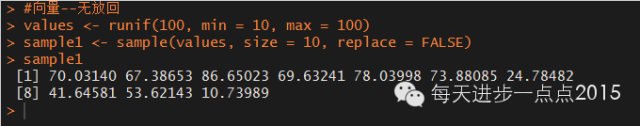#向量--有放回,不等概率
sample2 <- sample(c('A','B','C','D'), 500, replace = TRUE, prob = c(0.5,0.2,0.2,0.1))
table(sample2)
prop.table(table(sample2))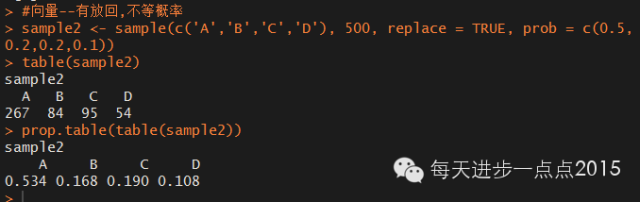#数据框
x <- runif(100)
y <- rnorm(100)
z <- rt(100,4)
df <- data.frame(x = x, y = y, z = z)

sample3 <- df[sample(1:nrow(df), size = 40),]
head(sample3)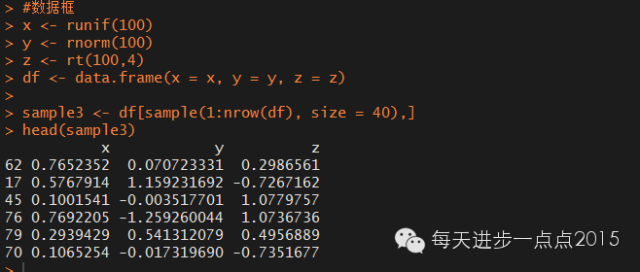二、系统抽样
系统抽样方法是一种简化的随机抽样法，最普遍的做法是从母群体的名单中，按照一定的间隔取出足够的个体组成样本。比如我们可以从这500家医院的名单中，每10间医院取一间来做为样本个体。但首先我们必须先随机决定一个起始的号码，也就是从1-10之间随机选出一个号码，假如选中的是3号，则我们从3号开始，每10号取一家医院做为样本(3, 13, 23, 33,…493)。关于系统抽样，在R中可以使用sampling包中的UPsystematic()函数实现，下面是UPsystematic()函数的语法及参数意义：

UPsystematic(pik,eps=1e-6)
pik：为一向量，存放抽样的包含概率
esp：为一控制值，默认为1e-6

例子：
{r}
x <- round(runif(1000, min = 1, max = 100))
#计算一阶包含概率
pik <- inclusionprobabilities(x,200)
#返回0-1值表示是否被抽样
s <- UPsystematic(pik)
head(getdata(x,s),10)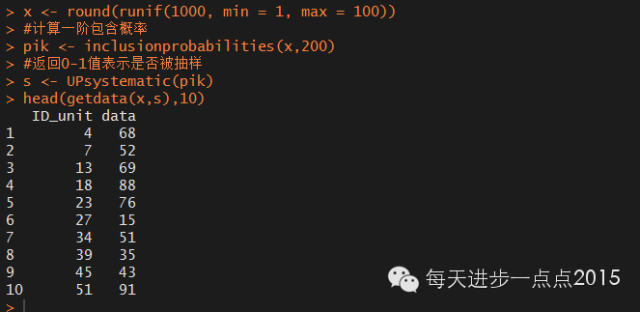但从上面的返回的ID_unit看，并不是系统抽样定义的那样，即等间隔的抽取样本。为保证与定义一致，这里自定义系统抽样的函数：
{r}
sys_sampling <- function(x, gap = 10, seed = 1234){
set.seed(seed)
i <- round(runif(1, min = 1, max = 10))
ID <- numeric()
sampling <- numeric()
while(i<=length(x)){
ID[ceiling(i/gap)] <- i
sampling[ceiling(i/gap)] <- x[i]
i <- i + gap
}
return(data.frame(ID = ID, data = sampling))
}

其中，x为待抽样的总体；gap为抽样间隔，默认为10；seed为种子数，用于从[1,10]之间随机挑选一个起始号设定随机种子，默认为1234。

例子：
{r}
head(sys_sampling(x = x, gap = 7, seed = 3),10)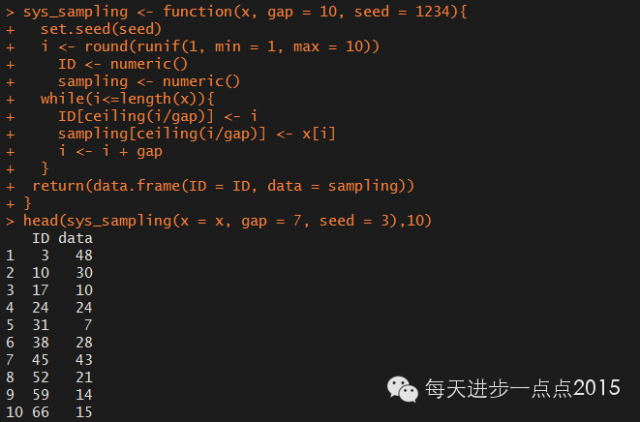三、分层抽样
分层或分组抽样是一种比SRS更精准的随机抽样法，所用的方法是跟据我们的研究性质，依照相关的条件把母群体中的个体分成不同的层别或组别(strata)，再分别从每一层别或组别中的个体随机抽出一定的个体来组成样本。在R中可以使用sampling包中的strata()函数实现，下面是strata()函数的语法及参数意义：

strata(data, stratanames=NULL, size,
method=c("srswor","srswr","poisson","systematic"),
pik,description=FALSE)
data：待抽样的数据框
stratanames：指定数据框中的分层变量
size：指定每个层中的抽样数量，默认按原数据中分层变量水平的顺序指定抽样数量
method：指定抽取各层数据的方法，默认为无放回的简单随机抽样，还可以是有放回的简单随机抽样、泊松抽样和系统抽样
pik：如果选择系统抽样时，需要指定系统抽样的包含概率pik向量

例子：
{r}

Stratified <- rep(c('A','B','C','D'), c(100,200,300,400))
Values <- round(runif(1000, min = 1, max = 1000))
df <- data.frame(Stratified = Stratified, Values = Values)

#等比例抽样
n <- 400
size <- round(400*table(df$Stratified)/length(df$Stratified))
s <- strata(data = df, stratanames = 'Stratified', size = size, method = 'srswor')
head(getdata(data = df, m = s))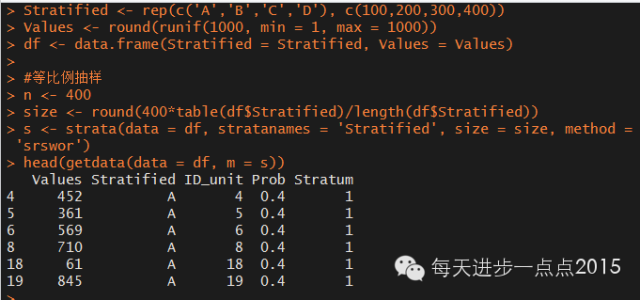#非等比例抽样，随意指定抽样数量
s <- strata(data = df, stratanames = 'Stratified', size = c(50, 100, 50, 200), method = 'srswor')
head(getdata(data = df, m = s))四、聚集抽样
聚集抽样也是跟据某种母群体的特性，将母群体中的个体分成不同的群组(clusters)，然后从这些群组中随机抽出部分的群组，再从被选中的群组中随机抽出足够的个体来组成样本。
从定义上看，聚集抽样与分层抽样很相似，但各自的组是完全两回事。在聚集抽样中，尽量保证组内数据差异特别大，而组间差异尽量小；在分层抽样中就恰恰相反，即组内差异尽量小，而组间差异要求很大。在R中可以使用sampling包中的cluster()函数实现，下面是cluster()函数的语法及参数意义：

cluster(data, clustername, size,
method=c("srswor","srswr","poisson","systematic"),
pik,description=FALSE)
data：待抽样的数据框
clustername：指定数据框中的聚集变量
size：指定抽取多少个组
method：指定抽取的方法，默认为无放回的简单随机抽样，还可以是有放回的简单随机抽样、泊松抽样和系统抽样
pik：如果选择系统抽样时，需要指定系统抽样的包含概率pik向量

例子：
{r}
Clusters <- rep(c('A','B','C','D','E','F','G'), c(100,200,300,400, 100, 200, 300))
Values <- round(runif(1600, min = 1, max = 1000))
df <- data.frame(Clusters = Clusters, Values = Values)

#从7个聚集组中随机抽取3个组
s <- cluster(data = df, clustername = 'Clusters', size = 3, method=c('srswor'))
#查看随机抽取了哪三个组
unique(sClusters) head(getdata(data = df, m = s))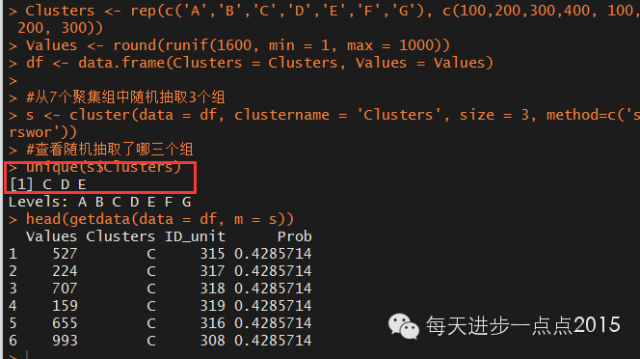展开全文• 针对红松母树林下刺五加资源，采用3不同抽样方法随机抽样、系统抽样和典型抽样，从数量估计、分布规律、地径结构和树高结构，以及地形因子对地径结构和树高结构的影响等个方面进行调查分析。结果表明：不同... • 对于运行不了次，一次运行不了多久的方法，我们不需要考虑性能优化，对于那些需要经常运行百次千次的方法，我们头脑里还是要性能这根弦。C#太优雅方便了，以至于很多人写程序时根本就把性能抛到脑后了，不...  对于运行不了几次，一次运行不了多久的方法，我们不需要考虑性能优化，对于那些需要经常运行几百次几千次的方法，我们头脑里还是要有性能这根弦。C#太优雅方便了，以至于很多人写程序时根本就把性能抛到脑后了，不愿意耗费心思去进行代码优化和算法优化，结果写出来的程序奇慢无比。不明真相的群众把这怪罪给C#语言。这不是C#的杯具，是程序员的无能。 2个月前，我研究sift（一种重要的图像分析算法）。最先找到了一个C#实现的library——libsift，这个library处理一张正常大小的图像，要耗时2-3分钟。后来，又找到一个C实现的library，处理同样的图像，耗时在1秒以内——秒杀。 昨天，我写Ransac（随机抽样一致性）算法代码时参考了libsift里的Ransac实现。不看不知道，一看吓一跳。那代码性能低下得无以复加。我随手优化了一下算法，就将随机抽样那部分的性能提高了上千倍。 下面详细道出。 一、Ransac Ransac是用途很广泛的算法，详细介绍请看http://en.wikipedia.org/wiki/RANSAC。下面简单介绍一下（没兴趣的可以略过不看）。 我们分析世界，需要对世界建模，把世界中的现象抽象成模型。每个模型，又存在一些参数，通过调节参数，可以得到不同的实例，进行推演。我们观察现象，得到一堆数据。如何为这堆数据找一个合适的模型，再确定合适的模型参数，这是很重要的问题，是人类理性的基础。 数据分两种：有效数据（inliers）和无效数据（outliers）。那些偏差不大的数据是有效数据，偏差大的数据是无效数据。 如果有效数据占大多数，无效数据只是很少量时，我们可以通过最小二乘法或类似的方法来确定模型的参数和误差。如果无效数据很多（比如，超过了50%的数据是无效数据），最小二乘法就失效了，我们需要新的算法。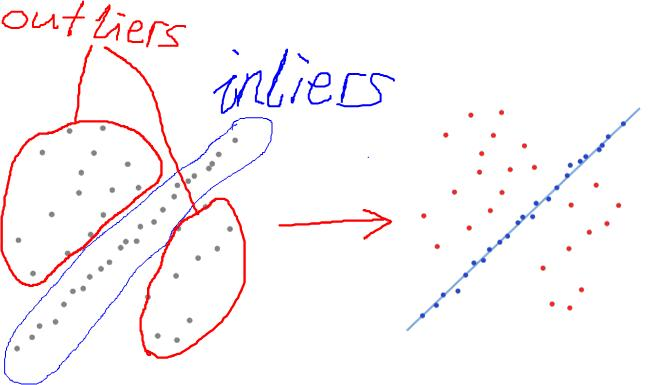上图左图是观察的数据。直觉可以看出，外面的散点是outliers，中间近似分布为一直线的是inliers。怎么设计一个算法，算出这条直线，使它对inliers的拟合度较高（如上图右图所示）？ 再举一个更直观的例子：上图左侧是一个验证码，我们将它看作“数据”。右侧是一个字符，我们将它看作“模型”，如何通过算法去除“数据”中的outlier，剩下inliner来和“模型”进行匹配 Ransac 是解决这类问题的代表性算法。它是一种随机算法，步骤如下： 二、libsift中Ransac算法的实现 Ransac算法中，model，model的拟合，不同参数model之间的比较都是因问题不同而不同，因此，可以将model抽象成接口。将model 抽象之后，Ransac 算法的骨干就只剩下一个随机采样的过程： 下面是libsift中Ransac算法的实现代码： 1 using System; 2 using System.Collections; 3 4 public class RANSAC 5 { 6 public interface IRANSACModel : ICloneable, IComparable 7 { 8 // Fit the model to the samples given. The number of samples is equal 9 // to or larger than the smallest number of points required for a fit 10 // ('n'). 11 // Return true if the fit can be done, false otherwise. 12 bool FitModel (ArrayList points); 13 14 // Return the fitting error of a single point against the current 15 // model. 16 double FittingErrorSingle (object point); 17 18 // Threshhold the given fit error of a point. 19 // Return true if the fitting error is small enough and the point is 20 // fitting. 21 // Return false if the point is not fitting. 22 bool ThreshholdPoint (double fitError); 23 24 // The overall fitting error of all points in FittingGround. This 25 // value is calculated by averaging all individual fitting errors of 26 // the points in the FittingGround. 27 double FittingErrorSum { 28 get; 29 set; 30 } 31 32 // All the points used to fit. Has to be set explicitly. 33 ArrayList FittingGround { 34 get; 35 set; 36 } 37 } 38 39 // Smallest number of points to be able to fit the model. 40 private int n; 41 42 // The number of iterations required. 43 private int k; 44 45 private RANSAC () 46 { 47 } 48 49 // n: Smallest number of points to be able to fit the model. 50 // k: The number of iterations required. 51 public RANSAC (int n, int k) 52 { 53 this.n = n; 54 this.k = k; 55 } 56 57 // ArrayList of Model's, sorted by summed fitting error. 58 // model: Model to fit 59 // points: List of point data to fit 60 // d: Number of nearby points required for a model to be accepted 61 public ArrayList FindModels (IRANSACModel model, ArrayList points, int d) 62 { 63 Random rand = new Random (); 64 ArrayList result = new ArrayList (); 65 66 if (points.Count < n) 67 throw (new ArgumentException 68 ("List of data is smaller than minimum fit requires.")); 69 70 for (int ki = 0 ; ki < k ; ++ki) { 71 ArrayList samples = new ArrayList (); 72 73 // Build random samples 74 for (int ri = 0 ; ri < n ; ++ri) { 75 object sampleToAdd; 76 sampleToAdd = points[rand.Next (0, points.Count)]; 77 78 if (samples.Contains (sampleToAdd)) 79 continue; 80 81 samples.Add (sampleToAdd); 82 } 83 84 if (model.FitModel (samples) == false) 85 continue; 86 87 ArrayList good = new ArrayList (); 88 double overAllFittingError = 0.0; 89 90 // Check all non-sample points for fit. 91 foreach (object point in points) { 92 if (samples.Contains (point)) 93 continue; 94 95 double fitError = model.FittingErrorSingle (point); 96 if (model.ThreshholdPoint (fitError)) { 97 good.Add (point); 98 overAllFittingError += fitError; 99 }100 }101 102 // good contains a list of all fitting points now. Check if there103 // are more than d points near our model.104 if (good.Count >= d) {105 good.AddRange (samples);106 IRANSACModel modelGood = (IRANSACModel) model.Clone ();107 108 modelGood.FitModel (good);109 modelGood.FittingErrorSum = overAllFittingError / good.Count;110 modelGood.FittingGround = good;111 112 result.Add (modelGood);113 }114 }115 result.Sort ();116 //Console.WriteLine ("got {0} modelfits", result.Count);117 118 return (result);119 }120 121 // Calculate the expected number of draws required when a fraction of122 // 'goodFraction' of the sample points is good and at least 'n' points are123 // required to fit the model. Add 'sdM' times the standard deviation to be124 // sure.125 // n: > 0126 // goodFraction: > 0.0 and <= 1.0127 // sdM: >= 0128 // return the guess for k, the expected number of draws.129 public static int GetKFromGoodfraction (int n, double goodFraction, int sdM)130 {131 double result;132 133 result = Math.Pow (goodFraction, -n);134 if (sdM > 0)135 result += sdM * Math.Sqrt (1.0 - Math.Pow (goodFraction, n));136 137 return ((int) (result + 0.5));138 }139 140 // Test Main141 public static void Main (string[] args)142 {143 Console.WriteLine ("n = 3, goodFraction = 0.3, sdM = 0: {0}",144 GetKFromGoodfraction (3, 0.3, 0));145 Console.WriteLine ("n = 3, goodFraction = 0.3, sdM = 10: {0}",146 GetKFromGoodfraction (3, 0.3, 10));147 }148 }149 150 不考虑Model部分，只考虑单次迭代过程中的随机抽样，可抽象出这样一个过程： 我把libsift的Ransac代码中上述逻辑部分单独提取出来了，并作了以下简化： 1 public class CaseLibSift 2 { 3 Random rand = new Random (); 4 5 public List<int> RandomSample(List<int> points, int n) 6 { 7 List<int> samples = new List<int>(); 8 9 // Build random samples10 for (int ri = 0; ri < n; ++ri)11 {12 int sampleToAdd;13 sampleToAdd = points[rand.Next(0, points.Count)];14 15 if (samples.Contains(sampleToAdd))16 continue;17 18 samples.Add(sampleToAdd);19 }20 21 // Check all non-sample points for fit.22 foreach (int point in points)23 {24 if (samples.Contains(point))25 continue;26 else27 samples.Add(point);28 }29 return samples;30 }31 } 准备测试数据，进行性能测试：Code <!-- Code highlighting produced by Actipro CodeHighlighter (freeware) http://www.CodeHighlighter.com/ --> 1 static int loops; 2 static int dataLength; 3 static int n; 4 5 static List<int> data; 6 7 static RandomSampleCompareCase() 8 { 9 loops = 50;10 dataLength = 10000;11 n = 4000;12 data = new List<int>(dataLength);13 for (int i = 0; i < dataLength; i++)14 data.Add(i);15 }16 17 public static void Test()18 {19 CaseLibSift c0 = new CaseLibSift();20 CodeTimer.Time("CaseLibSift", loops, () => { c0.RandomSample(data, n); });21 // CodeTimer.Time("MyCase", loops, () => { data.RandomSampleSplitOnSite(n); });22 Console.Read();23 } 这个测试中假设共有10000个数据，一共进行50次迭代，每次迭代的n值为4000。用老赵的CodeTimer测量运行时间，结果为： 24.5秒！雷人的慢！ 为什么会这样呢？主要问题出在这两句中： 您有更好的方案吗？ 四、我的方案 再回顾一下问题： 我采用的洗牌算法的变种。所谓洗牌问题，就是给定一个数组，编写程序将这个数组打乱。下面是一个经典的洗牌算法： 将上述洗牌算法稍微改变一下，就得到本文问题的答案： 这样，前n个元素就是随机取出的元素了。再考虑这样一个问题，就是n>N/2的情况，这时，n>N-n。我们不需要随机取出n个元素，只需要取出N-n个元素即可，剩下n个元素便是我们想要的随机采样结果。 把整个算法写成了扩展方法，代码如下：Code <!-- Code highlighting produced by Actipro CodeHighlighter (freeware) http://www.CodeHighlighter.com/ --> 1 /// <summary> 2 /// 代表IList中的一段[Start,End) 3 /// </summary> 4 /// <typeparam name="T"></typeparam> 5 public struct ListSegment<T> 6 { 7 public IList<T> Data; 8 public int Start; 9 public int End;10 }11 12 ……13 14 public static ListSegment<T> RandomSampleSplit<T>(this IList<T> data, int number)15 {16 IList<T> clone = new List<T>(data.Count);17 clone.AddRange(data);18 return clone.RandomSampleSplitOnSite(number);19 }20 21 public static ListSegment<T> RandomSampleSplitOnSite<T>(this IList<T> data, int number)22 {23 int count = data.Count;24 if (number < 1 || number >= count) throw new ArgumentException("number 必须大于 0 并且小于data中的元素数量。");25 26 int loops = number;27 28 if (number > (count >> 1)) // number 太大29 {30 loops = count - number;31 32 //从N个数中随机取出一个和最后一个元素交换,再从前面N-1个数中随机取一个和倒数第二个交换…33 for (int i = 0; i < loops; i++)34 {35 int index0 = Random.Next(0, count - i);36 int index1 = count - i - 1;37 T tmp = data[index0];38 data[index0] = data[index1];39 data[index1] = tmp;40 }41 }42 else43 {44 //从N个数中随机取出一个和第一个元素交换,再从后面N-1个数中随机取一个和第二个交换…45 for (int i = 0; i < loops; i++)46 {47 int index0 = Random.Next(i, count);48 int index1 = i;49 T tmp = data[index0];50 data[index0] = data[index1];51 data[index1] = tmp;52 }53 }54 55 ListSegment<T> seg = new ListSegment<T>();56 seg.Start = 0;57 seg.End = number;58 seg.Data = data;59 return seg;60 } 同CaseLibSift对比性能：Code <!-- Code highlighting produced by Actipro CodeHighlighter (freeware) http://www.CodeHighlighter.com/ -->1 public static void Test()2 {3 CaseLibSift c0 = new CaseLibSift();4 CodeTimer.Time("CaseLibSift", loops, () => { c0.RandomSample(data, n); });5 CodeTimer.Time("MyCase", loops, () => { data.RandomSampleSplitOnSite(n); });6 Console.Read();7 } 结果为： 对比可见，性能提高了千倍。 下面是我的Ransac完整实现代码：Code <!-- Code highlighting produced by Actipro CodeHighlighter (freeware) http://www.CodeHighlighter.com/ --> 1 public interface IRansacModel : ICollection<Vector>, ICloneable 2 { 3 double Error { get; } 4 void Update(); 5 bool FitPoint(Vector point); 6 /// <summary> 7 /// 比较IRansacModel的优劣。 8 /// </summary> 9 /// <param name="other"></param> 10 /// <returns></returns> 11 bool BestThan(IRansacModel other); 12 } 13 14 public abstract class RansacModelBase : List<Vector>, IRansacModel 15 { 16 public double Error { get; private set; } 17 18 public RansacModelBase():base() 19 { } 20 21 public RansacModelBase(int capacity):base(capacity) 22 { } 23 24 public abstract void Update(); 25 26 public abstract bool FitPoint(Vector point); 27 28 protected void CloneBaseFrom(RansacModelBase other) 29 { 30 this.Error = other.Error; 31 this.Clear(); 32 this.AddRange(other); 33 } 34 35 /// <summary> 36 /// 比较IRansacModel的优劣。 37 /// 默认情况下比较两者的 Error，Error 小则认为较优。 38 /// </summary> 39 /// <param name="other"></param> 40 /// <returns></returns> 41 public virtual bool BestThan(IRansacModel other) 42 { 43 return this.Error < other.Error; 44 } 45 46 #region ICloneable Members 47 48 public abstract object Clone(); 49 50 #endregion 51 52 } 53 54 public class Ransac<TModel> where TModel : IRansacModel 55 { 56 private int m_minNumberFitted; 57 private TModel m_model; 58 private Random m_rand = new Random(); 59 private int m_iteration; 60 61 private Ransac() 62 { 63 } 64 65 public Ransac(TModel model, int minNumberFitted, int iteration) 66 { 67 this.m_minNumberFitted = minNumberFitted; 68 this.m_iteration = iteration; 69 m_model = model; 70 } 71 72 public TModel Match(IList<Vector> points, int d) 73 { 74 if (points.Count < m_minNumberFitted) return default(TModel); 75 76 TModel bestModel = default(TModel); 77 78 for (int ki = 0; ki < m_iteration; ++ki) 79 { 80 TModel tmpModel = (TModel)this.m_model.Clone(); 81 82 // 随机采样 83 ListSegment<Vector> v = points.RandomSampleSplitOnSite(m_minNumberFitted); 84 85 for (int i = v.Start; i < v.End; i++) 86 { 87 tmpModel.Add(points[i]); 88 } 89 90 tmpModel.Update(); 91 92 IList<Vector> good = new List<Vector>(); 93 94 // Check all non-sample points for fit. 95 for (int i = v.End; i < points.Count; i++) 96 { 97 Vector point = points[i]; 98 if (tmpModel.FitPoint(point) == true) tmpModel.Add(point); 99 }100 101 if (tmpModel.Count >= d)102 {103 tmpModel.Update();104 if (bestModel == null) bestModel = tmpModel;105 else if (tmpModel.BestThan(bestModel)) bestModel = tmpModel;106 }107 }108 return (bestModel);109 }110 }  展开全文算法 loops library 测试 c# • 抽样方法： 一般方法： 随机抽样 直接从整体数据中等概率抽取n个样本。这种方法优势是，简单、好操作、适用于分布均匀的场景；缺点是总体大时无法一一编号 系统抽样 又称机械、等距抽样，将总体中个体按顺序... • 我们将介绍如何使用R软件实现常见的几种抽样方法，它们分别是：简单随机抽样、分层抽样、系统抽样、PPS抽样、整群抽样和多阶段抽样。下文的介绍中主要包含两方面的内容，抽样和估计。使用的软件是R-3.2.0。 进行抽样... • 分层抽样，即先将所有个体样本按照某种特征划分为个类别，然后从每个类别中使用随机抽样或等距抽样的方法选择个体组成样本。 分层抽样能明显的降低抽样误差，并且便于针对不同类别的数据样本进行单独研究，因此是... 分层抽样，即先将所有个体样本按照某种特征划分为几个类别，然后从每个类别中使用随机抽样或等距抽样的方法选择个体组成样本。 分层抽样能明显的降低抽样误差，并且便于针对不同类别的数据样本进行单独研究，因此是一种较好的实现方法。 该方法适用于带有分类逻辑的属性、标签等特征的数据 有数据如下：最后一列为分类标签，用0或者1来表示 导入包 import numpy as np import random  读取数据，并查看分类标签 data2 = np.loadtxt('data_preprocessing_data2.txt') # 导入带有分层逻辑的数据 each_sample_count = 20 # 定义每个分层的抽样数量 label_data_unique = np.unique(data2[:, -1]) # 定义分层值域 print(label_data_unique)#[0. 1.],数据一共被分为两个标签  遍历每个分层标签，用来做数据的分层划分，并且读取每条数据并判断数据的分层标签是否与分层标签相同，如果是则数据加入到各分层数据列表中 sample_data = [] # 定义空列表，用于存放最终抽样数据 sample_dict = {} # 定义空字典，用来显示各分层样本数量 for label_data in label_data_unique: # 遍历每个分层标签 sample_list = [] # 定义空列表，用于存放临时分层数据 for data_tmp in data2: # 读取每条数据 if data_tmp[-1] == label_data: # 如果数据最后一列等于标签 sample_list.append(data_tmp) # 将数据加入到分层数据中 each_sample_data = random.sample(sample_list, each_sample_count) # 对每层数据都随机抽样 sample_data.extend(each_sample_data) # 将抽样数据追加到总体样本集 sample_dict[label_data] = len(each_sample_data) # 样本集统计结果 print (sample_dict) # 打印输出样本集统计结果   展开全文python 数据分析 • 用EXCEL数据分析工具进行抽样 用EXCEL数据分析工具进行抽样有种方法第一举例EXCEL表格中一列3000多个数据我想在这3000多个数据中随机抽取200个出来3000多个都是文字的数据在一列里面要随机抽取出200个出来要... • 随机森林是一种有监督学习算法，是以决策树为基学习器的集成学习算法。随机森林非常简单，易于实现，计算开销也很小，但是它在分类和回归上表现出非常惊人的性能，因此，随机森林被誉为“代表集成学习技术水平的方法...  随机森林是一种有监督学习算法，是以决策树为基学习器的集成学习算法。随机森林非常简单，易于实现，计算开销也很小，但是它在分类和回归上表现出非常惊人的性能，因此，随机森林被誉为“代表集成学习技术水平的方法”。 一，随机森林的随机性体现在哪几个方面？ 1，数据集的随机选取 从原始的数据集中采取有放回的抽样（bagging），构造子数据集，子数据集的数据量是和原始数据集相同的。不同子数据集的元素可以重复，同一个子数据集中的元素也可以重复。 2，待选特征的随机选取 与数据集的随机选取类似，随机森林中的子树的每一个分裂过程并未用到所有的待选特征，而是从所有的待选特征中随机选取一定的特征，之后再在随机选取的特征中选取最优的特征 二，为什么使用随机森林？ 1，随机森林既可以用于分类问题，也可以用于回归问题 2，过拟合是个关键的问题，可能会让模型的结果变得糟糕，但是对于随机森林来说，如果随机森林的树足够多，那么分类器就不会过拟合模型 3，随机森林分类器可以处理缺失值 4，随机森林分类器可以用分类值建模 三，随机森林的构建过程 1，从原始训练集中使用Bootstraping方法随机有放回采样取出m个样本，共进行n_tree次采样。生成n_tree个训练集 2，对n_tree个训练集，我们分别训练n_tree个决策树模型 3，对于单个决策树模型，假设训练样本特征的个数为n，那么每次分裂时根据信息增益/信息增益比/基尼指数 选择最好的特征进行分裂 4，每棵树都已知这样分裂下去，知道该节点的所有训练样例都属于同一类。在决策树的分裂过程中不需要剪枝 5，将生成的多颗决策树组成随机森林。对于分类问题，按照多棵树分类器投票决定最终分类结果；对于回归问题，由多颗树预测值的均值决定最终预测结果 注意：OOB（out-of-bag ）：每棵决策树的生成都需要自助采样，这时就有1/3的数据未被选中，这部分数据就称为袋外数据。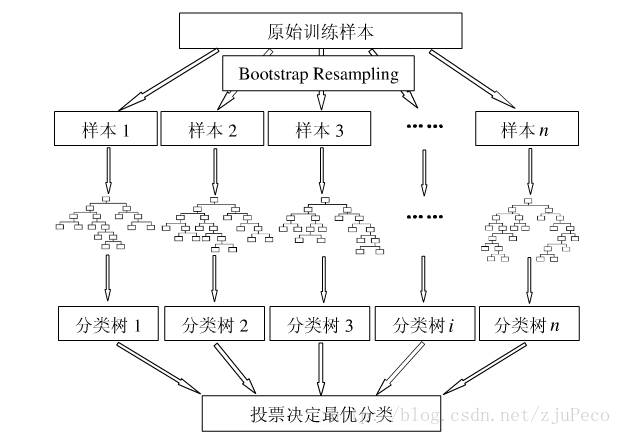四，随机森林优缺点总结 优点 由于采用了集成算法，本身精度比大多数单个算法要好，所以准确性高 在测试集上表现良好，由于两个随机性的引入，使得随机森林不容易陷入过拟合（样本随机，特征随机） 在工业上，由于两个随机性的引入，使得随机森林具有一定的抗噪声能力，对比其他算法具有一定优势 由于树的组合，使得随机森林可以处理非线性数据，本身属于非线性分类（拟合）模型 它能够处理很高维度（feature很多）的数据，并且不用做特征选择，对数据集的适应能力强：既能处理离散型数据，也能处理连续型数据，数据集无需规范化 训练速度快，可以运用在大规模数据集上 可以处理缺省值（单独作为一类），不用额外处理 由于有袋外数据（OOB），可以在模型生成过程中取得真实误差的无偏估计，且不损失训练数据量 在训练过程中，能够检测到feature间的互相影响，且可以得出feature的重要性，具有一定参考意义 由于每棵树可以独立、同时生成，容易做成并行化方法 由于实现简单、精度高、抗过拟合能力强，当面对非线性数据时，适于作为基准模型 缺点 当随机森林中的决策树个数很多时，训练时需要的空间和时间会比较大 随机森林中还有许多不好解释的地方，有点算是黑盒模型 在某些噪音比较大的样本集上，RF的模型容易陷入过拟合 五，特征重要性评估 现实情况下，一个数据集中往往有成百上千个特征，如何在其中选择比结果影响最大的那几个特征，以此来缩减建立模型时特征数是我们比较关心的问题。这样的方法其实很多，比如主成分分析，lasso等等。不过这里我们学习的是用随机森林来进行特征筛选。 用随机森林进行特征重要性评估的思想就是看每个特征在随机森林中的每棵树上做了多大的贡献，然后取个平均值，最后比一比特征之间的贡献大小。 贡献大小通常使用基尼指数（Gini index）或者袋外数据（OOB）错误率作为评估指标来衡量。这里我们再学习一下基尼指数来评价的方法。 我们将变量重要性评分（variable importance measures）用VIM来表示，将Gini指数用GI来表示，假设m个特征X1，X2，X3，......Xc，现在要计算出每个特征Xj的Gini指数评分VIMj（Gini）,亦即第j个特征在RF所有决策树中节点分裂不纯度的平均改变量。 Gini指数的计算公式为：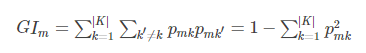其中，K表示有K个类别。Pmk表示节点m中类列k所占的比例。 直观的说，就是随便从节点m中随机抽取两个样本，其类别标记不一致的概率。 特征Xj在节点m的重要性，即节点m分支前后的Gini指数变化量为：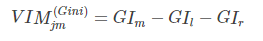其中，GIl和GIr分别表示分枝后两个新节点的Gini指数。 如果，特征Xj在决策树i中出现的节点在集合M中，那么Xj在第i颗树的重要性为：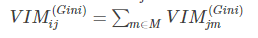假设RF中共有n颗树，那么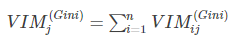最后，把所有求得的重要性评分做一个归一化处理即可。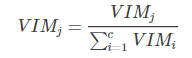5.1 特征选择 5.1.1 特征选择的步骤 在特征重要性的基础上，特征选择的步骤如下： 计算每个特征的重要性，并按降序排序 确定要剔除的比例，依据特征重要性剔除相应比例的特征，得到一个新的特征集 用新的特征集重复上述过程，直到剩下m个特征（m为提前设定的值） 根据上述代码中得到的各个特征集合特征集对应的袋外误差率，选择袋外误差率最低的特征集 5.1.2 特征重要性的估计方法 特征重要性的估计通常有两种方法：一是使用uniform或者gaussian抽取随机值替换原特征；一是通过permutation的方式将原来的所有N个样本的第i个特征值重新打乱分布，第二种方法更加科学，保证了特征替代值与原特征的分布是近似的。这种方法叫做permutation test ，即在计算第i个特征的重要性的时候，将N 个特征的第i个特征重新洗牌，然后比较D和表现的差异性，如果差异很大，则表明第i个特征是重要的。 5.2 示例——利用随机森林进行特征选择，然后使用SVR进行训练 1，利用随机森林进行特征选择 代码： import numpy as np import pandas as pd from sklearn.ensemble import RandomForestClassifier # url = 'http://archive.ics.uci.edu/ml/machine-learning-databases/wine/wine.data' url1 = pd.read_csv(r'wine.txt',header=None) # url1 = pd.DataFrame(url1) # df = pd.read_csv(url1,header=None) url1.columns = ['Class label', 'Alcohol', 'Malic acid', 'Ash', 'Alcalinity of ash', 'Magnesium', 'Total phenols', 'Flavanoids', 'Nonflavanoid phenols', 'Proanthocyanins', 'Color intensity', 'Hue', 'OD280/OD315 of diluted wines', 'Proline'] # print(url1) # 查看几个标签 # Class_label = np.unique(url1['Class label']) # print(Class_label) # 查看数据信息 # info_url = url1.info() # print(info_url) # 除去标签之外，共有13个特征，数据集的大小为178， # 下面将数据集分为训练集和测试集 from sklearn.model_selection import train_test_split print(type(url1)) # url1 = url1.values # x = url1[:,0] # y = url1[:,1:] x,y = url1.iloc[:,1:].values,url1.iloc[:,0].values x_train,x_test,y_train,y_test = train_test_split(x,y,test_size=0.3,random_state=0) feat_labels = url1.columns[1:] # n_estimators：森林中树的数量 # n_jobs 整数 可选（默认=1） 适合和预测并行运行的作业数，如果为-1，则将作业数设置为核心数 forest = RandomForestClassifier(n_estimators=10000, random_state=0, n_jobs=-1) forest.fit(x_train, y_train) # 下面对训练好的随机森林，完成重要性评估 # feature_importances_ 可以调取关于特征重要程度 importances = forest.feature_importances_ print("重要性：",importances) x_columns = url1.columns[1:] indices = np.argsort(importances)[::-1] for f in range(x_train.shape): # 对于最后需要逆序排序，我认为是做了类似决策树回溯的取值，从叶子收敛 # 到根，根部重要程度高于叶子。 print("%2d) %-*s %f" % (f + 1, 30, feat_labels[indices[f]], importances[indices[f]])) # 筛选变量（选择重要性比较高的变量） threshold = 0.15 x_selected = x_train[:,importances > threshold] # 可视化 import matplotlib.pyplot as plt plt.figure(figsize=(10,6)) plt.title("红酒的数据集中各个特征的重要程度",fontsize = 18) plt.ylabel("import level",fontsize = 15,rotation=90) plt.rcParams['font.sans-serif'] = ["SimHei"] plt.rcParams['axes.unicode_minus'] = False for i in range(x_columns.shape): plt.bar(i,importances[indices[i]],color='orange',align='center') plt.xticks(np.arange(x_columns.shape),x_columns,rotation=90,fontsize=15) plt.show() 结果： RangeIndex: 178 entries, 0 to 177 Data columns (total 14 columns): Class label 178 non-null int64 Alcohol 178 non-null float64 Malic acid 178 non-null float64 Ash 178 non-null float64 Alcalinity of ash 178 non-null float64 Magnesium 178 non-null int64 Total phenols 178 non-null float64 Flavanoids 178 non-null float64 Nonflavanoid phenols 178 non-null float64 Proanthocyanins 178 non-null float64 Color intensity 178 non-null float64 Hue 178 non-null float64 OD280/OD315 of diluted wines 178 non-null float64 Proline 178 non-null int64 dtypes: float64(11), int64(3) memory usage: 19.5 KB 重要性： [0.10658906 0.02539968 0.01391619 0.03203319 0.02207807 0.0607176 0.15094795 0.01464516 0.02235112 0.18248262 0.07824279 0.1319868 0.15860977] 1) Color intensity 0.182483 2) Proline 0.158610 3) Flavanoids 0.150948 4) OD280/OD315 of diluted wines 0.131987 5) Alcohol 0.106589 6) Hue 0.078243 7) Total phenols 0.060718 8) Alcalinity of ash 0.032033 9) Malic acid 0.025400 10) Proanthocyanins 0.022351 11) Magnesium 0.022078 12) Nonflavanoid phenols 0.014645 13) Ash 0.013916 图：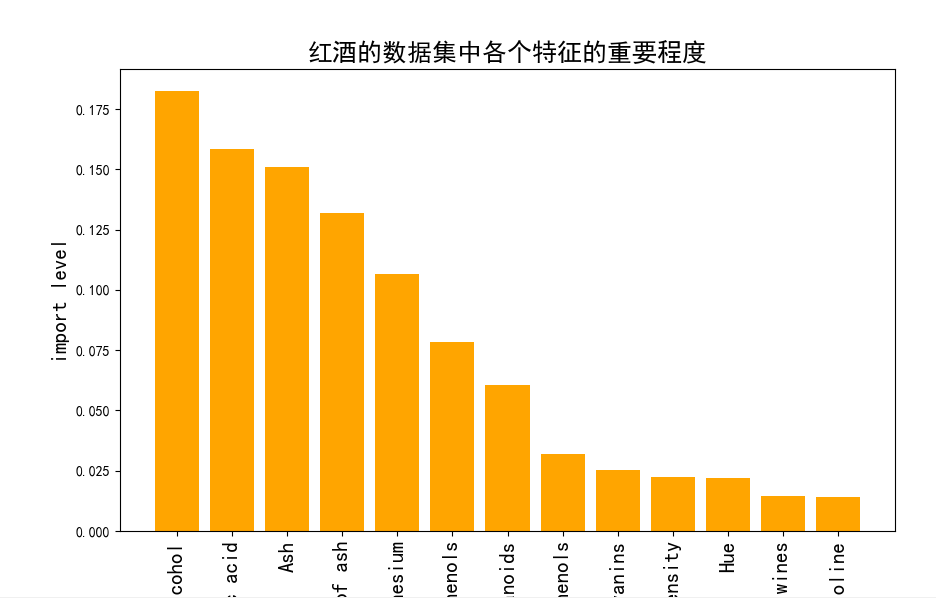2，利用SVR进行训练 代码： from sklearn.svm import SVR # SVM中的回归算法 import pandas as pd from sklearn.model_selection import train_test_split import matplotlib.pyplot as plt import numpy as np # 数据预处理，使得数据更加有效的被模型或者评估器识别 from sklearn import preprocessing from sklearn.externals import joblib # 获取数据 origin_data = pd.read_csv('wine.txt',header=None) X = origin_data.iloc[:,1:].values Y = origin_data.iloc[:,0].values print(type(Y)) # print(type(Y.values)) # 总特征 按照特征的重要性排序的所有特征 all_feature = [ 9, 12, 6, 11, 0, 10, 5, 3, 1, 8, 4, 7, 2] # 这里我们选取前三个特征 topN_feature = all_feature[:3] print(topN_feature) # 获取重要特征的数据 data_X = X[:,topN_feature] # 将每个特征值归一化到一个固定范围 # 原始数据标准化，为了加速收敛 # 最小最大规范化对原始数据进行线性变换，变换到[0,1]区间 data_X = preprocessing.MinMaxScaler().fit_transform(data_X) # 利用train_test_split 进行训练集和测试集进行分开 X_train,X_test,y_train,y_test = train_test_split(data_X,Y,test_size=0.3) # 通过多种模型预测 model_svr1 = SVR(kernel='rbf',C=50,max_iter=10000) # 训练 # model_svr1.fit(data_X,Y) model_svr1.fit(X_train,y_train) # 得分 score = model_svr1.score(X_test,y_test) print(score) 结果： 0.8211850237886935 scikit-learn随机森林类库概述 sklearn.ensemble模块包含了两种基于随机决策树的平均算法：RandomForest算法和Extra-Trees算法。这两种算法都采用了很流行的树设计思想：perturb-and-combine思想。这种方法会在分类器的构建时，通过引入随机化，创建一组各不一样（diverse）的分类器。这种ensemble方法的预测会给出各个分类器预测的平均。 在sklearn.ensemble库中，我们可以找到Random Forest分类和回归的实现：RandomForestClassifier和RandomForestRegression 有了这些模型后，我们的做法是立马上手操作，因为学习中提供的示例都很简单，但是实际中遇到很多问题，下面概述一下： 命名模型调教的很好了，可是效果离我们的想象总有些偏差？——模型训练的第一步就是要定要目标，往错误的方向走太多也是后退。 凭直觉调了某个参数，可是居然没有任何作用，有时甚至起到反作用？——定好目标后，接下来就是要确定哪些参数是影响目标的，其对目标是正影响还是负影响，影响的大小。 感觉训练结束遥遥无期，sklearn只是一个在小数据上的玩具？——虽然sklearn并不是基于分布式计算环境而设计的，但是我们还是可以通过某些策略提高训练的效率 模型开始训练了，但是训练到哪一步了呢？——饱暖思淫欲啊，目标，性能和效率都得了满足后，我们有时还需要有别的追求，例如训练过程的输出，袋外得分计算等等。 在scikit-learn中，RF的分类类是RandomForestClassifier，回归类是RandomForestRegressor。当然RF的变种Extra Trees也有，分类类ExtraTreesClassifier，回归类ExtraTreesRegressor。由于RF和Extra Trees的区别较小，调参方法基本相同，本文只关注于RF的调参。 RandomForests 在随机森林（RF）中，该ensemble方法中的每棵树都基于一个通过可放回抽样（boostrap）得到的训练集构建。另外，在构建树的过程中，当split一个节点时，split的选择不再是对所有features的最佳选择。相反的，在features的子集中随机进行split反倒是最好的split方式。这种随机的后果是，整个forest的bias，从而得到一个更好的模型。 sklearn的随机森林（RF）实现通过对各分类结果预测求平均得到，而非让每个分类器进行投票（vote）。 Ext-Trees 在Ext-Trees中(详见ExtraTreesClassifier和 ExtraTreesRegressor)，该方法中，随机性在划分时会更进一步进行计算。在随机森林中，会使用侯选feature的一个随机子集，而非查找最好的阈值，对于每个候选feature来说，阈值是抽取的，选择这种随机生成阈值的方式作为划分原则。通常情况下，在减小模型的variance的同时，适当增加bias是允许的。 首先看一个类的参数： class sklearn.ensemble.RandomForestClassifier(n_estimators=10, criterion='gini', max_depth=None, min_samples_split=2, min_samples_leaf=1, min_weight_fraction_leaf=0.0, max_features=’auto’, max_leaf_nodes=None, min_impurity_decrease=0.0, min_impurity_split=None, bootstrap=True, oob_score=False, n_jobs=1, random_state=None, verbose=0, warm_start=False, class_weight=None) 一，RF Bagging框架参数 和GBDT对比，GBDT的框架参数比较多，重要的有最大迭代器个数，步长和子采样比例，调参起来比较费力。但是RF则比较简单，这是因为Bagging框架里的各个弱学习器之间是没有依赖关系的，这减小调参的难度，换句话说，达到同样的调参效果，RF调参数时间要比GBDT少一些， 下面我来看看RF重要的Bagging框架的参数，由于RandomForestClassifier和RandomForestRegressor参数绝大部分相同，这里会将它们一起讲，不同点会指出。 1) n_estimators: 也就是弱学习器的最大迭代次数，或者说最大的弱学习器的个数。一般来说n_estimators太小，容易欠拟合，n_estimators太大，又容易过拟合，一般选择一个适中的数值。RandomForestClassifier和RandomForestRegressor默认是10。在实际调参的过程中，我们常常将n_estimators和下面介绍的参数learning_rate一起考虑。 2) oob_score :即是否采用袋外样本来评估模型的好坏。默认识False。有放回采样中大约36.8%的没有被采样到的数据，我们常常称之为袋外数据（Out Of Bag 简称OOB），这些数据没有参与训练集模型的拟合，因此可以用来检测模型的泛化能力。个人推荐设置为True，因为袋外分数反应了一个模型拟合后的泛化能力。 3) criterion: 即CART树做划分时对特征的评价标准。分类模型和回归模型的损失函数是不一样的。分类RF对应的CART分类树默认是基尼系数gini,另一个可选择的标准是信息增益。回归RF对应的CART回归树默认是均方差mse，另一个可以选择的标准是绝对值差mae。一般来说选择默认的标准就已经很好的。 4）bootstrap：默认是True，是否有放回的采样。 5）verbose：日志亢长度，int表示亢长度，o表示输出训练过程，1表示偶尔输出 ，>1表示对每个子模型都输出 从上面可以看出， RF重要的框架参数比较少，主要需要关注的是 n_estimators，即RF最大的决策树个数。当使用这些方法的时候，最主要的参数是调整n_estimators和max_features。n_estimators指的是森林中树的个数，树数目越大越好，但是会增加计算开销，另外，注意如果超过限定数量后，计算将会停止。 二，RF决策树参数 下面我们再来看RF的决策树参数，它要调参的参数基本和GBDT相同，如下： 1) RF划分时考虑的最大特征数max_features: 可以使用很多种类型的值，默认是"None",意味着划分时考虑所有的特征数；如果是"log2"意味着划分时最多考虑个特征；如果是"sqrt"或者"auto"意味着划分时最多考虑个特征。如果是整数，代表考虑的特征绝对数。如果是浮点数，代表考虑特征百分比，即考虑（百分比xN）取整后的特征数。其中N为样本总特征数。一般来说，如果样本特征数不多，比如小于50，我们用默认的"None"就可以了，如果特征数非常多，我们可以灵活使用刚才描述的其他取值来控制划分时考虑的最大特征数，以控制决策树的生成时间。max_features指的是，当划分一个节点的时候，features的随机子集的size，该值越小，variance会变小，但是bais会变大。（int 表示个数，float表示占所有特征的百分比，auto表示所有特征数的开方，sqrt表示所有特征数的开放，log2表示所有特征数的log2值，None表示等于所有特征数） 2) 决策树最大深度max_depth: 默认可以不输入，如果不输入的话，决策树在建立子树的时候不会限制子树的深度。一般来说，数据少或者特征少的时候可以不管这个值。如果模型样本量多，特征也多的情况下，推荐限制这个最大深度，具体的取值取决于数据的分布。常用的可以取值10-100之间。（int表示深度，None表示树会生长到所有叶子都分到一个类，或者某节点所代表的样本已小于min_samples_split） 3) 内部节点再划分所需最小样本数min_samples_split: 这个值限制了子树继续划分的条件，如果某节点的样本数少于min_samples_split，则不会继续再尝试选择最优特征来进行划分。 默认是2.如果样本量不大，不需要管这个值。如果样本量数量级非常大，则推荐增大这个值。（int表示样本数，2表示默认值） 4) 叶子节点最少样本数min_samples_leaf: 这个值限制了叶子节点最少的样本数，如果某叶子节点数目小于样本数，则会和兄弟节点一起被剪枝。 默认是1,可以输入最少的样本数的整数，或者最少样本数占样本总数的百分比。如果样本量不大，不需要管这个值。如果样本量数量级非常大，则推荐增大这个值。 5）叶子节点最小的样本权重和min_weight_fraction_leaf：这个值限制了叶子节点所有样本权重和的最小值，如果小于这个值，则会和兄弟节点一起被剪枝。 默认是0，就是不考虑权重问题。一般来说，如果我们有较多样本有缺失值，或者分类树样本的分布类别偏差很大，就会引入样本权重，这时我们就要注意这个值了。 6) 最大叶子节点数max_leaf_nodes: 通过限制最大叶子节点数，可以防止过拟合，默认是"None”，即不限制最大的叶子节点数。如果加了限制，算法会建立在最大叶子节点数内最优的决策树。如果特征不多，可以不考虑这个值，但是如果特征分成多的话，可以加以限制，具体的值可以通过交叉验证得到。 7) 节点划分最小不纯度min_impurity_split: 这个值限制了决策树的增长，如果某节点的不纯度(基于基尼系数，均方差)小于这个阈值，则该节点不再生成子节点。即为叶子节点 。一般不推荐改动默认值1e-7。 8）用于拟合和预测的并行运行的工作数量n_jobs：一般取整数，可选的（默认值为1），如果为-1，那么工作数量被设置为核的数量，机器上所有的核都会被使用（跟CPU核数一致）。如果n_jobs=k，则计算被划分为k个job，并运行在K核上。注意，由于进程间通信的开销，加速效果并不会是线性的（job数K不会提示K倍）通过构建大量的树，比起单颗树所需要的时间，性能也能得到很大的提升， 9）随机数生成器random_state：随机数生成器使用的种子，如果是RandomState实例，则random_stats就是随机数生成器；如果为None，则随机数生成器是np.random使用的RandomState实例。 上面决策树参数中最重要的包括最大特征数max_features， 最大深度max_depth， 内部节点再划分所需最小样本数min_samples_split和叶子节点最少样本数min_samples_leaf。 根据经验 对于回归问题：好的缺省值max_features = n_features; 对于分类问题：好的缺省值是max_features=sqrt(n_features)。n_features指的是数据中的feature总数。 当设置max_depth=None，以及min_samples_split=1时，通常会得到好的结果（完全展开的树）。但需要注意，这些值通常不是最优的，并且会浪费RAM内存。最好的参数应通过cross-validation给出。另外需要注意： 在随机森林中，缺省时会使用bootstrap进行样本抽样(bootstrap=True) ； 而extra-trees中，缺省策略为不使用bootstrap抽样 (bootstrap=False)； 当使用bootstrap样本时，泛化误差可能在估计时落在out-of-bag样本中。此时，可以通过设置oob_score=True来开启。 三，如何调参呢？ 参数分类的目的在于缩小调参的范围，首先我们要明确训练的目标，把目标类的参数定下来。接下来，我们需要根据数据集的大小，考虑是否采用一些提高训练效率的策略，否则一次训练就三天三夜，时间太久了，所以我们需要调整哪些影响整体的模型性能的参数。 1，调参的目标：偏差和方差的协调 偏差和方差通过准确率来影响着模型的性能。调参的目标就是为了达到整体模型的偏差和方差的大和谐！进一步，这些参数又可以分为两类：过程影响类及子模型影响类。在子模型不变的前提下，某些参数可以通过改变训练的过程，从而影响着模型的性能，诸如：“子模型数”（n_estimators），“学习率”（learning_rate）等，另外，我们还可以通过改变子模型性能来影响整体模型的性能，诸如：“最大树深度”（max_depth），‘分裂条件’（criterion）等。正由于bagging的训练过程旨在降低方差，而Boosting的训练过程旨在降低偏差，过程影响类的参数能够引起整体模型性能的大幅度变化。一般来说，在此前提下，我们继续微调子模型影响类的参数，从而进一步提高模型的性能。 2，参数对整体模型性能的影响 假设模型是一个多元函数F，其输出值为模型的准确度。我们可以固定其他参数，从而对某个参数整体模型性能的影响进行分析：是正影响还是负影响，影响的单调性？ 对Random Forest来说，增加“子模型树”（n_estimators）可以明显降低整体模型的方差，且不会对子模型的偏差和方差有任何影响。模型的准确度会随着“子模型数”的增加而提高，由于减少的是整体模型方差公式的第二项，故准确度的提高有一个上线。在不同的场景下，“分裂条件”（criterion）对模型的准确度的影响也不一样，该参数需要在实际运行时灵活调整。调整“最大叶子节点数”（max_leaf_models）以及“最大树深度”（max_depth）之一，可以粗粒度地调整树的结构：叶节点越多或者树越深，意味着子模型的偏差月底，方差越高；同时，调整”分裂所需要最小样本数”（min_samples_split），“叶节点最小样本数”（min_samples_leaf）及“叶节点最小权重总值”（min_weight_fraction_leaf），可以更细粒度地调整树的结构：分裂所需样本数越少或者叶节点所需样本越少，也意味着子模型越复杂。一般来说，我们总采用bootstrap对样本进行子采样来降低子模型之间的关联度，从而降低整体模型的方差。适当地减少“分裂时考虑的最大特征数”（max_features），给子模型注入了另外的随机性，同样也达到了降低子模型之间关联度的效果。但是一味地降低该参数也是不行的，因为分裂时可选特征变少，模型的偏差会越来越大。在下图中，我们可以看到这些参数对Random Forest整体模型性能的影响：3，一个朴实的方案：贪心的坐标下降法 到此为止，我们终于知道需要调整哪些参数，对于单个参数，我们也知道怎么调整才能提升性能。然后，表示模型的函数F并不是一元函数，这些参数需要共同调参才能得到全局最优解。也就是说，把这些参数丢给调参算法（诸如Grid Search）？对于小数据集，我们还能这么任性，但是参数组合爆炸，在大数据集上，实际上网格搜索也不一定能得到全局最优解。 坐标下降法是一类优化算法，其最大的优势在于不同计算待优化的目标函数的梯度。我们最容易想到一种特别朴实的类似于坐标下降法的方法，与坐标下降法不同的是，其不同循环使用各个参数进行调整，而是贪心地选取了对整体模型性能影响最大的参数。参数对整体模型性能的影响力是动态变化的，故每一轮坐标选取的过程中，这种方法在对每个坐标的下降方向进行一次直线搜索（line search）。首先，找到那些能够提升整体模型性能的参数，其次确保提升是单调或者近似单调。这意味着，我们筛选出来的参数是整体模型性能有正影响的，且这种影响不是偶然性的，要知道，训练过程的随机性也会导致整体模型性能的细微区别，而这种区别是不具有单调性的。最后，在这些筛选出来的参数中，选取影响最大的参数进行调整即可。 无法对整体模型性能进行量化，也就谈不上去比较参数影响整体模型性能的程度，是的，我们还没有一个准确的方法来量化整体模型性能，只能通过交叉验证来近似计算整体模型性能。然而交叉验证也存在随机性，假设我们以验证集上的平均准确度作为整体模型的准确度，我们还得关心在各个验证集上准确度的变异系数，如果变异系数过大，则平均值作为整体模型的准确率也是不合适的。在接下来的案例分析中，我们所谈及的整体模型性能均是指平均准确度。 4，调参步骤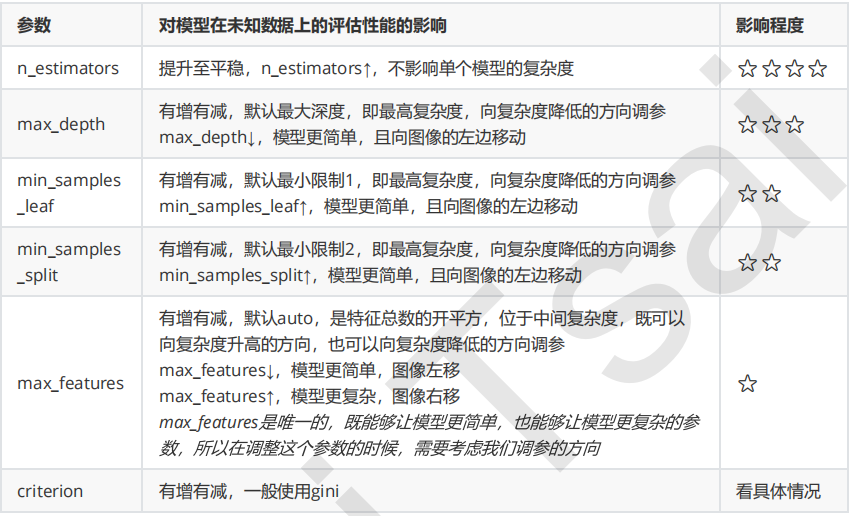1，首先先调既不会增加模型复杂度，又对模型影响最大的参数n_estimators（学习曲线） 2，找到最佳值后，调max_depth（单个网格搜索，也可以使用学习曲线） 一般根据数据的大小来进行一个探视，当数据集很小的时候，可以采用1~10，或者1~20这样的试探，但是对于大型数据来说，我们应该尝试30~50 层深度（或许更深） 3，接下来依次对各个参数进行调参 注意：对大型数据集，max_leaf_nodes可以尝试从1000来构建，先输入1000，每100个叶子一个区间，再逐渐缩小范围 对于min_samples_split 和 min_samples_leaf，一般从他们的最小值开始向上增加10或者20，面对高纬度高样本数据，如果不放心可以直接50+，对于大型数据可能需要200~300的范围，如果调整的时候发现准确率无论如何都上不来，可以放心大胆的调试一个很大的数据，大力限制模型的复杂度。 5，一个使用随机森林回归算法的调参实例 如果想看完整数据及其代码：请点击我 5.1 使用gridsearchcv探索n_estimators的最佳值 def random_forest_parameter_tuning1(feature_data, label_data, test_feature): from sklearn.ensemble import RandomForestRegressor from sklearn.model_selection import train_test_split from sklearn.metrics import mean_squared_error from sklearn.model_selection import GridSearchCV X_train, X_test, y_train, y_test = train_test_split(feature_data, label_data, test_size=0.23) param_test1 = { 'n_estimators': range(10, 71, 10) } model = GridSearchCV(estimator=RandomForestRegressor( min_samples_split=100, min_samples_leaf=20, max_depth=8, max_features='sqrt', random_state=10), param_grid=param_test1, cv=5 ) model.fit(X_train, y_train) # 对测试集进行预测 y_pred = model.predict(X_test) # 计算准确率 MSE = mean_squared_error(y_test, y_pred) RMSE = np.sqrt(MSE) print(RMSE) return model.best_score_, model.best_params_ 结果如下： {'n_estimators': 70} 0.6573670183811001 这样我们得到了最佳的弱学习器迭代次数，为70.。 5.2 对决策树最大深度 max_depth 和内部节点再划分所需要的最小样本数求最佳值 我们首先得到了最佳弱学习器迭代次数，接着我们对决策树最大深度max_depth和内部节点再划分所需要最小样本数min_samples_split进行网格搜索。 def random_forest_parameter_tuning2(feature_data, label_data, test_feature): from sklearn.ensemble import RandomForestRegressor from sklearn.model_selection import train_test_split from sklearn.metrics import mean_squared_error from sklearn.model_selection import GridSearchCV X_train, X_test, y_train, y_test = train_test_split(feature_data, label_data, test_size=0.23) param_test2 = { 'max_depth': range(3, 14, 2), 'min_samples_split': range(50, 201, 20) } model = GridSearchCV(estimator=RandomForestRegressor( n_estimators=70, min_samples_leaf=20, max_features='sqrt', oob_score=True, random_state=10), param_grid=param_test2, cv=5 ) model.fit(X_train, y_train) # 对测试集进行预测 y_pred = model.predict(X_test) # 计算准确率 MSE = mean_squared_error(y_test, y_pred) RMSE = np.sqrt(MSE) print(RMSE) return model.best_score_, model.best_params_ 结果为： {'max_depth': 13, 'min_samples_split': 50} 0.7107311632187736 对于内部节点再划分所需要最小样本数min_samples_split，我们暂时不能一起定下来，因为这个还和决策树其他的参数存在关联。 5.3 求内部节点再划分所需要的最小样本数min_samples_split和叶子节点最小样本数min_samples_leaf的最佳参数 下面我们对内部节点在划分所需要最小样本数min_samples_split和叶子节点最小样本数min_samples_leaf一起调参。 def random_forest_parameter_tuning3(feature_data, label_data, test_feature): from sklearn.ensemble import RandomForestRegressor from sklearn.model_selection import train_test_split from sklearn.metrics import mean_squared_error from sklearn.model_selection import GridSearchCV X_train, X_test, y_train, y_test = train_test_split(feature_data, label_data, test_size=0.23) param_test3 = { 'min_samples_split': range(10, 90, 20), 'min_samples_leaf': range(10, 60, 10), } model = GridSearchCV(estimator=RandomForestRegressor( n_estimators=70, max_depth=13, max_features='sqrt', oob_score=True, random_state=10), param_grid=param_test3, cv=5 ) model.fit(X_train, y_train) # 对测试集进行预测 y_pred = model.predict(X_test) # 计算准确率 MSE = mean_squared_error(y_test, y_pred) RMSE = np.sqrt(MSE) print(RMSE) return model.best_score_, model.best_params_ 结果如下： {'min_samples_leaf': 10, 'min_samples_split': 10} 0.7648492269870218 5.4 求最大特征数max_features的最佳参数 def random_forest_parameter_tuning4(feature_data, label_data, test_feature): from sklearn.ensemble import RandomForestRegressor from sklearn.model_selection import train_test_split from sklearn.metrics import mean_squared_error from sklearn.model_selection import GridSearchCV X_train, X_test, y_train, y_test = train_test_split(feature_data, label_data, test_size=0.23) param_test3 = { 'max_features': range(3, 9, 2), } model = GridSearchCV(estimator=RandomForestRegressor( n_estimators=70, max_depth=13, min_samples_split=10, min_samples_leaf=10, oob_score=True, random_state=10), param_grid=param_test3, cv=5 ) model.fit(X_train, y_train) # 对测试集进行预测 y_pred = model.predict(X_test) # 计算准确率 MSE = mean_squared_error(y_test, y_pred) RMSE = np.sqrt(MSE) print(RMSE) return model.best_score_, model.best_params_ 结果如下： {'max_features': 7} 0.881211719251515 5.5 汇总出我们搜索到的最佳参数，然后训练 def random_forest_train(feature_data, label_data, test_feature, submitfile): from sklearn.ensemble import RandomForestRegressor from sklearn.model_selection import train_test_split from sklearn.metrics import mean_squared_error X_train, X_test, y_train, y_test = train_test_split(feature_data, label_data, test_size=0.23) params = { 'n_estimators': 70, 'max_depth': 13, 'min_samples_split': 10, 'min_samples_leaf': 10, 'max_features': 7 } model = RandomForestRegressor(**params) model.fit(X_train, y_train) # 对测试集进行预测 y_pred = model.predict(X_test) # 计算准确率 MSE = mean_squared_error(y_test, y_pred) RMSE = np.sqrt(MSE) print(RMSE) submit = pd.read_csv(submitfile) submit['y'] = model.predict(test_feature) submit.to_csv('my_random_forest_prediction1.csv', index=False) 四，Random Forest 调参示例：Digit Recognizer 在这里，我们选取Kaggle上101教学赛的Digit Recognizer作为案例来演示对RandomForestClassifier调参的过程。当然，我们也不要傻乎乎地手工去设定不同的参数，然后训练模型，借助sklearn.grid_search库中的GridSearchCV类，不仅可以自动化调参，同时还可以对每一种参数组合进行交叉验证计算平均准确度。 4.1 例子： >>> from sklearn.ensemble import RandomForestClassifier >>> from sklearn.datasets import make_classification >>> >>> X, y = make_classification(n_samples=1000, n_features=4, ... n_informative=2, n_redundant=0, ... random_state=0, shuffle=False) >>> clf = RandomForestClassifier(max_depth=2, random_state=0) >>> clf.fit(X, y) RandomForestClassifier(bootstrap=True, class_weight=None, criterion='gini', max_depth=2, max_features='auto', max_leaf_nodes=None, min_impurity_decrease=0.0, min_impurity_split=None, min_samples_leaf=1, min_samples_split=2, min_weight_fraction_leaf=0.0, n_estimators=10, n_jobs=1, oob_score=False, random_state=0, verbose=0, warm_start=False) >>> print(clf.feature_importances_) [ 0.17287856 0.80608704 0.01884792 0.00218648] >>> print(clf.predict([[0, 0, 0, 0]]))  4.2 方法如下：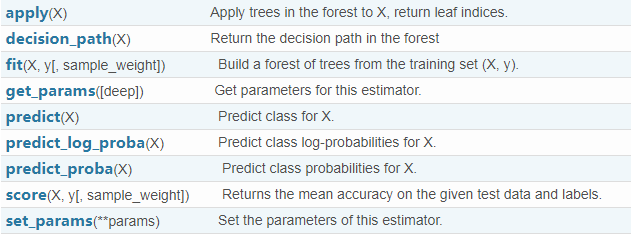五，进行预测的几种常用的方法 1 ） predict_proba(x) : 给出带有概率值的结果。每个点在所有label（类别）的概率和为1. 2） predict(x)： 直接给出预测结果，内部还是调用的predict_proba()。根据概率的结果看哪个类型的预测值最高就是那个类型。 3）predict_log_proba(x)： 和predict_proba基本上一样，只是把结果做了log()处理。 >>> from sklearn.cross_validation import cross_val_score >>> from sklearn.datasets import make_blobs >>> from sklearn.ensemble import RandomForestClassifier >>> from sklearn.ensemble import ExtraTreesClassifier >>> from sklearn.tree import DecisionTreeClassifier >>> X, y = make_blobs(n_samples=10000, n_features=10, centers=100, ... random_state=0) >>> clf = DecisionTreeClassifier(max_depth=None, min_samples_split=1, ... random_state=0) >>> scores = cross_val_score(clf, X, y) >>> scores.mean() 0.97... >>> clf = RandomForestClassifier(n_estimators=10, max_depth=None, ... min_samples_split=1, random_state=0) >>> scores = cross_val_score(clf, X, y) >>> scores.mean() 0.999... >>> clf = ExtraTreesClassifier(n_estimators=10, max_depth=None, ... min_samples_split=1, random_state=0) >>> scores = cross_val_score(clf, X, y) >>> scores.mean() > 0.999 True 六，随机森林分类算法的实现 代码： #_*_coding:UTF_8_*_ # 导入需要导入的库 import pandas as pd import numpy as np import math from sklearn.ensemble import RandomForestClassifier from sklearn.ensemble import ExtraTreesClassifier from sklearn.tree import DecisionTreeClassifier from sklearn import model_selection ,metrics from sklearn.model_selection import cross_val_score from sklearn.model_selection import train_test_split import matplotlib.pyplot as plt import matplotlib as mpl from sklearn.datasets import make_blobs import warnings # 忽略一些版本不兼容等警告 warnings.filterwarnings("ignore") # 每个样本有几个属性或者特征 n_features = 2 x,y = make_blobs(n_samples=300,n_features=n_features,centers=6) x_train,x_test,y_train,y_test = train_test_split(x,y,random_state=1,train_size=0.7) # 绘制样本显示 # plt.scatter(x[:,0],x[:,1],c=y) # plt.show() # 传统决策树，随机森林算法 极端随机数的区别 DT = DecisionTreeClassifier(max_depth=None,min_samples_split=2,random_state=0) RF = RandomForestClassifier(n_estimators=10,max_features=math.sqrt(n_features), max_depth=None,min_samples_split=2,bootstrap=True) EC = ExtraTreesClassifier(n_estimators=10,max_features=math.sqrt(n_features), max_depth=None,min_samples_split=2,bootstrap=False) # 训练 DT.fit(x_train,y_train) RF.fit(x_train,y_train) EC.fit(x_train,y_train) #区域预测 # 第0列的范围 x1_min,x1_max = x[:,0].min(),x[:,0].max() # 第1列的范围 x2_min,x2_max = x[:,1].min(),x[:,1].max() # 生成网格采样点行列均为200点 x1,x2 = np.mgrid[x1_min:x1_max:200j,x2_min:x2_max:200j] # 将区域划分为一系列测试点用去学习的模型预测，进而根据预测结果画区域 area_sample_point = np.stack((x1.flat,x2.flat),axis=1) # 所有区域点进行预测 area1_predict = DT.predict(area_sample_point) area1_predict = area1_predict.reshape(x1.shape) area2_predict = RF.predict(area_sample_point) area2_predict = area2_predict.reshape(x1.shape) area3_predict = EC.predict(area_sample_point) area3_predict = area3_predict.reshape(x1.shape) # 用来正常显示中文标签 mpl.rcParams['font.sans-serif'] = [u'SimHei'] # 用来正常显示负号 mpl.rcParams['axes.unicode_minus'] = False # 区域颜色 classifier_area_color = mpl.colors.ListedColormap(['#A0FFA0','#FFA0A0','#A0A0FF']) # 样本所属类别颜色 cm_dark = mpl.colors.ListedColormap(['r','g','b']) # 绘图 # 第一个子图 plt.subplot(2,2,1) plt.pcolormesh(x1,x2,area1_predict,cmap = classifier_area_color) plt.scatter(x_train[:,0],x_train[:,1],c =y_train,marker='o',s=50,cmap=cm_dark) plt.scatter(x_test[:,0],x_test[:,1],c =y_test,marker='x',s=50,cmap=cm_dark) plt.xlabel('data_x',fontsize=8) plt.ylabel('data_y',fontsize=8) plt.xlim(x1_min,x1_max) plt.ylim(x2_min,x2_max) plt.title(u'DecisionTreeClassifier: 传统决策树',fontsize=8) plt.text(x1_max-9,x2_max-2,u'o-------train ; x--------test')

# 第二个子图
plt.subplot(2,2,2)

plt.pcolormesh(x1,x2,area2_predict,cmap = classifier_area_color)
plt.scatter(x_train[:,0],x_train[:,1],c =y_train,marker='o',s=50,cmap=cm_dark)
plt.scatter(x_test[:,0],x_test[:,1],c =y_test,marker='x',s=50,cmap=cm_dark)

plt.xlabel('data_x',fontsize=8)
plt.ylabel('data_y',fontsize=8)
plt.xlim(x1_min,x1_max)
plt.ylim(x2_min,x2_max)
plt.title(u'RandomForestClassifier: 随机森林算法',fontsize=8)
plt.text(x1_max-9,x2_max-2,u'o-------train ; x--------test$') # 第三个子图 plt.subplot(2,2,3) plt.pcolormesh(x1,x2,area3_predict,cmap = classifier_area_color) plt.scatter(x_train[:,0],x_train[:,1],c =y_train,marker='o',s=50,cmap=cm_dark) plt.scatter(x_test[:,0],x_test[:,1],c =y_test,marker='x',s=50,cmap=cm_dark) plt.xlabel('data_x',fontsize=8) plt.ylabel('data_y',fontsize=8) plt.xlim(x1_min,x1_max) plt.ylim(x2_min,x2_max) plt.title(u'ExtraTreesClassifier: 极端随机树',fontsize=8) plt.text(x1_max-9,x2_max-2,u'o-------train ; x--------test$')

# 第四个子图
plt.subplot(2,2,4)
y = []
# 交叉验证
score_DT = cross_val_score(DT,x_train,y_train)
y.append(score_DT.mean())
score_RF = cross_val_score(RF,x_train,y_train)
y.append(score_RF.mean())
score_EC = cross_val_score(EC,x_train,y_train)
y.append(score_EC.mean())

print('DecisionTreeClassifier交叉验证准确率为:'+str(score_DT.mean()))
print('RandomForestClassifier交叉验证准确率为:'+str(score_RF.mean()))
print('ExtraTreesClassifier交叉验证准确率为:'+str(score_EC.mean()))

x = [0,1,2]
plt.bar(x,y,0.4,color='green')
plt.xlabel("0--DecisionTreeClassifier;1--RandomForestClassifier;2--ExtraTreesClassifie", fontsize=8)
plt.ylabel("平均准确率", fontsize=8)
plt.ylim(0.9, 0.99)
plt.title("交叉验证", fontsize=8)
for a, b in zip(x, y):
plt.text(a, b, b, ha='center', va='bottom', fontsize=10)

plt.show()

结果：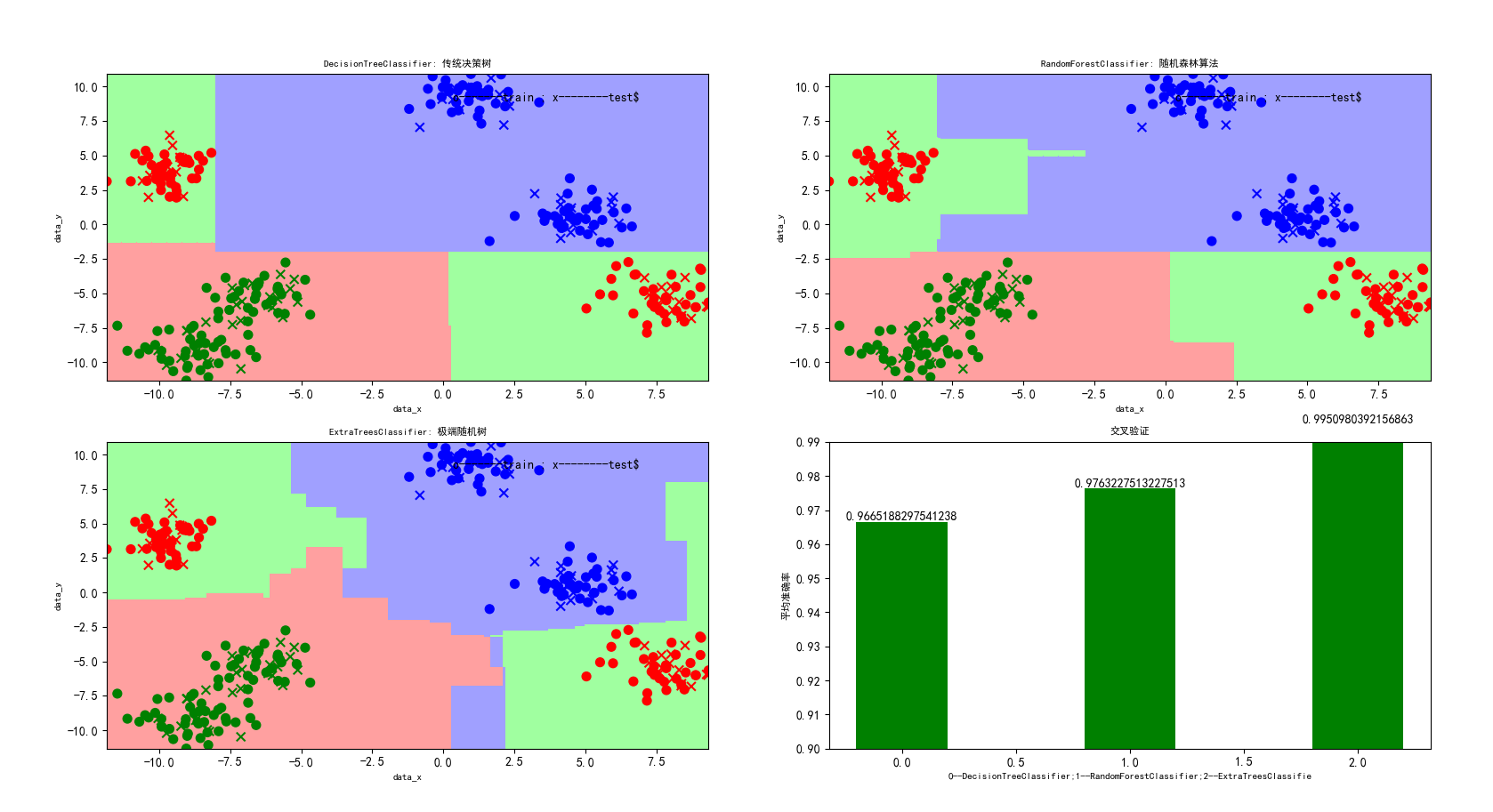七，随机森林回归算法的实现

代码：

#随机森林回归
import matplotlib as mpl
import numpy as np
import warnings
import matplotlib.pyplot as plt
from sklearn.tree import DecisionTreeRegressor
from sklearn.ensemble import RandomForestRegressor
from sklearn.ensemble import ExtraTreesRegressor

#忽略一些版本不兼容等警告
warnings.filterwarnings("ignore")

#产生心状坐标
t = np.arange(0,2*np.pi,0.1)
x = 16*np.sin(t)**3
x=x[:, np.newaxis]
y = 13*np.cos(t)-5*np.cos(2*t)-2*np.cos(3*t)-np.cos(4*t)
y[::7]+= 3* (1 - np.random.rand(9))                      #增加噪声，在每数2个数的时候增加一点噪声

#传统决策树线性回归,随机森林回归，极端森林回归
rf1=DecisionTreeRegressor()
rf2=RandomForestRegressor(n_estimators=1000)           #一般来说n_estimators越大越好，运行结果呈现出的两种结果该值分别是10和1000
rf3=ExtraTreesRegressor()

#三种算法的预测
y_rf1 =rf1.fit(x,y).predict(x)
y_rf2 =rf2.fit(x,y).predict(x)
y_rf3 =rf3.fit(x,y).predict(x)

#为了后面plt.text定位
x1_min, x1_max = x[:].min(), x[:].max()
x2_min, x2_max = y[:].min(), y[:].max()

mpl.rcParams['font.sans-serif'] = [u'SimHei']            #用来正常显示中文标签
mpl.rcParams['axes.unicode_minus'] = False

plt.scatter(x, y, color='darkorange', label='data')
plt.hold('on')

plt.plot(x, y_rf1, color='b',  label='DecisionTreeRegressor')
plt.plot(x, y_rf2, color='g',  label='RandomForestRegressor')
plt.plot(x, y_rf3, color='r',  label='ExtraTreesRegressor')

plt.xlabel('data_x')
plt.ylabel('data_y')
plt.title('python_machine-learning_RandomForest(n_estimators=1000)-----心状学习')
plt.legend()
plt.text(x1_max-4, x2_max-1, u'$o---Sample-Point$')
plt.show()

结果：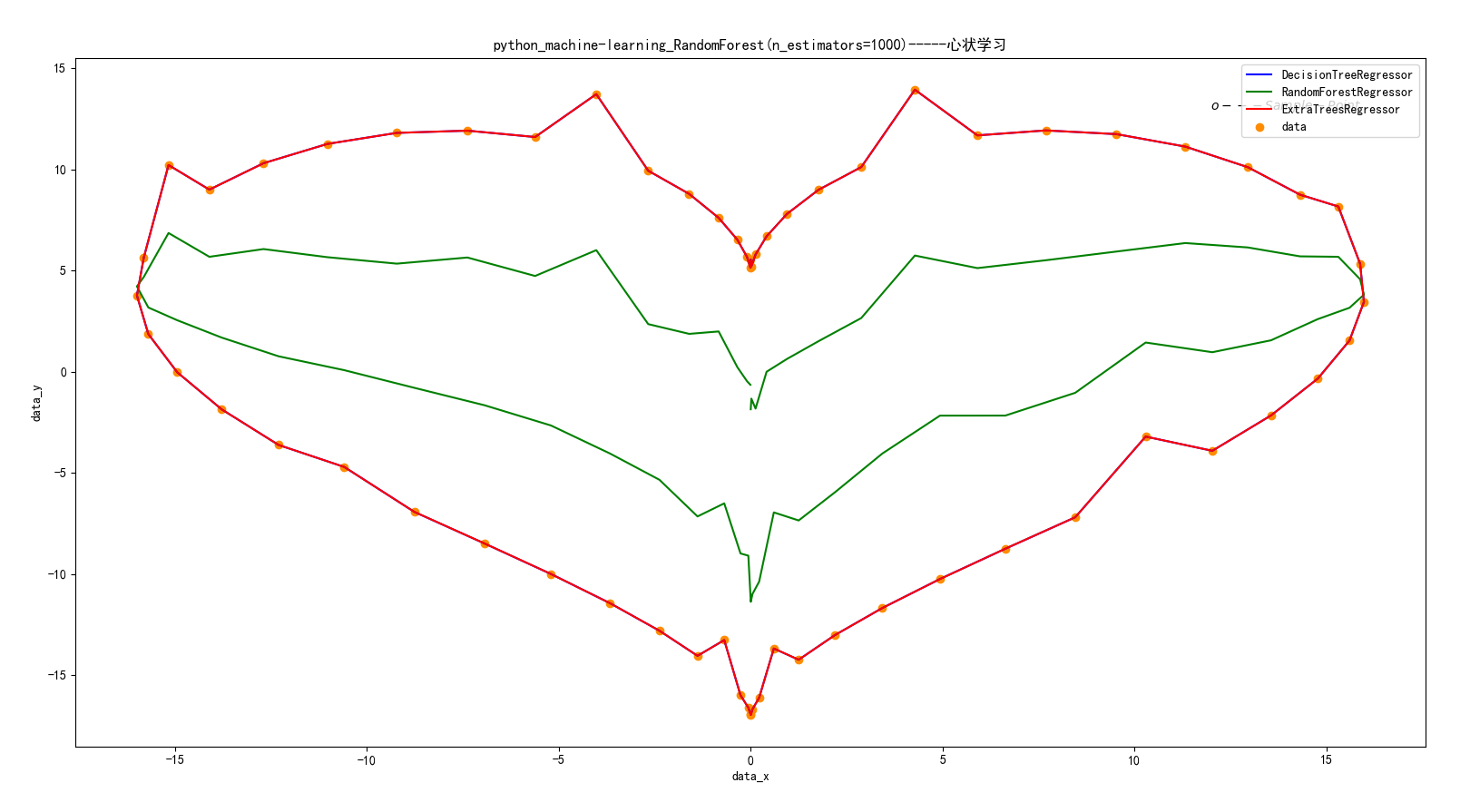八，随机森林分类算法其他机器学习分类算法进行对比

代码：

import numpy as np
import matplotlib.pyplot as plt
from matplotlib.colors import ListedColormap
from sklearn.cross_validation import train_test_split
from sklearn.preprocessing import StandardScaler
from sklearn.datasets import make_moons, make_circles, make_classification
from sklearn.neighbors import KNeighborsClassifier
from sklearn.svm import SVC
from sklearn.tree import DecisionTreeClassifier
from sklearn.ensemble import RandomForestClassifier, AdaBoostClassifier
from sklearn.naive_bayes import GaussianNB
from sklearn.lda import LDA
from sklearn.qda import QDA

h = .02  # step size in the mesh

names = ["Nearest Neighbors", "Linear SVM", "RBF SVM", "Decision Tree",
"Random Forest", "AdaBoost", "Naive Bayes", "LDA", "QDA"]
classifiers = [
KNeighborsClassifier(3),
SVC(kernel="linear", C=0.025),
SVC(gamma=2, C=1),
DecisionTreeClassifier(max_depth=5),
RandomForestClassifier(max_depth=5, n_estimators=10, max_features=1),
AdaBoostClassifier(),
GaussianNB(),
LDA(),
QDA()]

X, y = make_classification(n_features=2, n_redundant=0, n_informative=2,
random_state=1, n_clusters_per_class=1)
rng = np.random.RandomState(2)
X += 2 * rng.uniform(size=X.shape)
linearly_separable = (X, y)

datasets = [make_moons(noise=0.3, random_state=0),
make_circles(noise=0.2, factor=0.5, random_state=1),
linearly_separable
]

figure = plt.figure(figsize=(27, 9))
i = 1
# iterate over datasets
for ds in datasets:
# preprocess dataset, split into training and test part
X, y = ds
X = StandardScaler().fit_transform(X)
X_train, X_test, y_train, y_test = train_test_split(X, y, test_size=.4)

x_min, x_max = X[:, 0].min() - .5, X[:, 0].max() + .5
y_min, y_max = X[:, 1].min() - .5, X[:, 1].max() + .5
xx, yy = np.meshgrid(np.arange(x_min, x_max, h),
np.arange(y_min, y_max, h))

# just plot the dataset first
cm = plt.cm.RdBu
cm_bright = ListedColormap(['#FF0000', '#0000FF'])
ax = plt.subplot(len(datasets), len(classifiers) + 1, i)
# Plot the training points
ax.scatter(X_train[:, 0], X_train[:, 1], c=y_train, cmap=cm_bright)
# and testing points
ax.scatter(X_test[:, 0], X_test[:, 1], c=y_test, cmap=cm_bright, alpha=0.6)
ax.set_xlim(xx.min(), xx.max())
ax.set_ylim(yy.min(), yy.max())
ax.set_xticks(())
ax.set_yticks(())
i += 1

# iterate over classifiers
for name, clf in zip(names, classifiers):
ax = plt.subplot(len(datasets), len(classifiers) + 1, i)
clf.fit(X_train, y_train)
score = clf.score(X_test, y_test)

# Plot the decision boundary. For that, we will assign a color to each
# point in the mesh [x_min, m_max]x[y_min, y_max].
if hasattr(clf, "decision_function"):
Z = clf.decision_function(np.c_[xx.ravel(), yy.ravel()])
else:
Z = clf.predict_proba(np.c_[xx.ravel(), yy.ravel()])[:, 1]

# Put the result into a color plot
Z = Z.reshape(xx.shape)
ax.contourf(xx, yy, Z, cmap=cm, alpha=.8)

# Plot also the training points
ax.scatter(X_train[:, 0], X_train[:, 1], c=y_train, cmap=cm_bright)
# and testing points
ax.scatter(X_test[:, 0], X_test[:, 1], c=y_test, cmap=cm_bright,
alpha=0.6)

ax.set_xlim(xx.min(), xx.max())
ax.set_ylim(yy.min(), yy.max())
ax.set_xticks(())
ax.set_yticks(())
ax.set_title(name)
ax.text(xx.max() - .3, yy.min() + .3, ('%.2f' % score).lstrip('0'),
size=15, horizontalalignment='right')
i += 1

figure.subplots_adjust(left=.02, right=.98)
plt.show()

结果：这里随机生成了三个样本集，分割面近似为月形、圆形和线形的。我们可以重点对比一下决策树和随机森林对样本空间的分割：

1）从准确率上可以看出，随机森林在这三个测试集上都要优于单棵决策树，90%>85%，82%>80%，95%=95%；

2）从特征空间上直观地可以看出，随机森林比决策树拥有更强的分割能力（非线性拟合能力）。

九，解决问题：在调用sklearn时出现 Unknown label type: 'unknown' 或者'continuous'

在sklearn模型训练出现如下错误：

‘ValueError: Unknown label type: ‘unknown’

解决方法：以GBDT为例：train_y后加上astype(‘int’)即可

gbdt.fit(train_x,train_y.astype(‘int’))

集成学习：https://scikit-learn.org/dev/modules/ensemble.html

https://www.jianshu.com/p/a779f0686acc

https://blog.csdn.net/qq547276542/article/details/78304454

https://blog.csdn.net/sun_shengyun/article/details/54618121

https://blog.csdn.net/zjuPeco/article/details/77371645?locationNum=7&fps=1

https://www.cnblogs.com/simpleDi/p/9979818.html

转载https://www.cnblogs.com/wj-1314/p/9628303.html

展开全文• 　随机森林是一种有监督学习算法，是以决策树为基学习器的集成学习算法。随机森林非常简单，易于实现，计算开销也很小，但是它在分类和回归上表现出非常惊人的性能，因此，随机森林被誉为“代表集成学习技术水平的...机器学习 Python
• 　随机森林是一种有监督学习算法，是以决策树为基学习器的集成学习算法。随机森林非常简单，易于实现，计算开销也很小，但是它在分类和回归上表现出非常惊人的性能，因此，随机森林被誉为“代表集成学习技术水平的...
• 来源 | 博客园作者 | 战争热诚随机森林是一种有监督学习算法，是以决策树为基学习器的集成学习算法。随机森林非常简单，易于实现，计算开销也很小，但是它在分类和回归上表现出非常惊人的性能，因此，随机森林被誉为...
• 随机数在数值计算,随机抽样等很多领域重要作用,尤其在密码学及信息安全领域中成为一个重要的研究方向,在数字加密,密钥获取以及身份验证等方面更是重中之重。 随机数Number分为伪随机数和真随机数,现阶段的伪随机...matlab 随机数
• 与其像常规引导程序那样随机抽样（替换）每个观测值，不如对这些块进行随机抽样。 这样，在每个块中都保留了时间依赖性。 块引导程序的问题在于对块长度或将时间序列分解为块的数量的选择具有很高的敏感性。 {...
• 4.11随机抽样 4.12抽样分布 4.13二项分布的正态逼近 4.14Minitab指令 4.15小结 重要公式 补充练习 第五部分数据分析:中心值方差和比例 第五章关于总体中心值的推断 5.1引言和案例 5.2μ的估计 5.3估计μ...
• 尽管针对顶级提供商的抽样方法可能具有成本效益，但不一定能提供信息质量，因为它忽略了价格和数据速率的变化，而这仅是小型提供商也可以满足的包容性方法。 这项研究在两个方面是新颖的。 它提出了一采样技术，...
• 2.1数据的来源 （1）数据的直接来源：调查数据和实验数据 （2）数据的间接来源 ... 调查实践中经常采用的概率抽样方式以下几种：a.简单随机抽样；b.分层抽样；c.整群抽样；d.系统从抽样；e.多阶段抽样。 ...
• 一、总体与样本定义 总体：所研究的所有事件的...① 简单随机抽样：抽签，随机编号生成器，放回抽样和不放回两 ② 分层抽样：将总体分为个层，层内部相似，层与层之间差距较大，再对每一层进行简单随机抽样...中心极限定理
•  五种抽样方法：  1、简单随机：选取热量相同且每个样本同等概率被选择的样本  2、系统：简单的系统抽取样本  3、任意：使用一个碰巧很容易被选择的样本  4、整群：先将总体分为不同组群，从中随机挑选个组...统计学
• 前面几章的介绍了几种分类算法，当然各有优缺。如果将这些不同的分类器组合起来，就构成了我们今天要介绍的集成方法或者说元算法。集成方法有多种形式：可以使多种算法的集成，也可以是一种算法在不同设置下的集成，...
• Matlab关于蒙特卡洛仿真资料讲义和程序举例-第二讲-第五讲.rar 看到有些同学在找这方面的资料，的确...下面简单介绍几种产生随机数的常用数学方法。 复制代码 Matlab1.jpg 蒙特卡洛仿真资料matlab
• RANSAC是“RANdom SAmple Consensus（随机抽样一致）”的缩写。它可以从一组包含“局外点”的观测数据集中，通过迭代方式估计数学模型的参数。它是一不确定的算法——它一定的概率得出一个合理的结果；为了提高...
• 例如：北京方便面官方发布一袋北京方便面重100g（默认是正态分布），为了证明官方是否说谎，我们随机从刚刚批发进货来的箱北京方便面中，随机抽样一袋，来证明。这里我们就用假设检验方法来证明（实则是用反证法）...假设检验 分布拟合检验
• 比如说迁移前后的数据条数是否一致，数据是否一致，这个时候怎么办呢，验证条数还好说，要是验证数据是否一致呢，对于重要的数据当然要每条都不会差错，随机抽样验证肯定是不行的，万一遗漏了就麻烦了，而且两张表...
• 31.解读学习曲线：其他情况 下图反映了高方差，通过增加数据集可以改善。 下图反映了高偏差和高方差，需要找到一...（1）与其只使用10个样本训练单个模型，不如从你原来的100个样本中进行随机有放回抽样，选择...
• Bagging算法中，弱分类器的生成可以以下几种：基于样本分布的弱分类器生成，可以根据样本分布抽样产生，也可以不抽样，在弱分类中应用样本分布（这种方法需要修改弱分类器的实现过程）；基于属性选择的弱分类器...